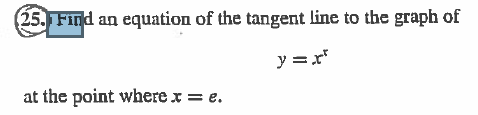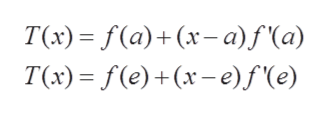(25. Find an equation of the tangent line to the graph ofy rat the point where x = e.

Question

How do I do this?help_outlineImage Transcriptionclose(25. Find an equation of the tangent line to the graph of y r at the point where x = e. fullscreen
Step 1

We are given a function and at a point

Step 2

We can use tangent line formula

and then we can plug a=ehelp_outlineImage TranscriptioncloseT(x) — F(a)+ (x-а) f(а) T(x) (e)(x-e)f'(e) fullscreen
Step 3

Firstly, we will find f...

Want to see the full answer?

See Solution

Want to see this answer and more?

Our solutions are written by experts, many with advanced degrees, and available 24/7

See Solution
Tagged in

Derivative